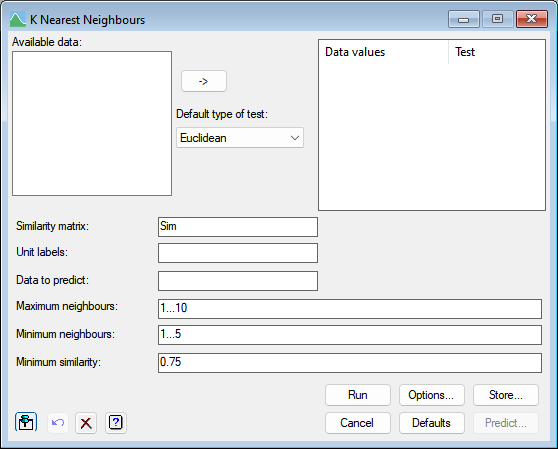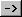1. Home
2. K Nearest Neighbours

# K Nearest Neighbours

Select menu Stats | Multivariate analysis | K Nearest Neighbours.

This menu can be used to classify items or predict their responses by examining their k nearest neighbours. To do this it forms a similarity matrix from a set of variables (factors or variates) and then uses this with the KNNTRAIN procedure to optimize the options of the k nearest neighbour algorithm for predicting an outcome variable given in the Data to predict field. These are the minimum and maximum number of neighbours and the minimum similarity.

Stats | Multivariate analysis | K Nearest Neighbours.
2. Fill in the fields as required then click Run.The similarity coefficient that is calculated allows variables to be qualitative, quantitative or dichotomous, or mixtures of these types; values of some of the variables may be missing for some samples. The values of a similarity coefficient vary between zero and unity: two samples have a similarity of unity only when both have identical values for all variables; a value of zero occurs when the values for the two samples differ maximally for all variables. Items must have a similarity with the observation being predicted of at least Minimum similarity to be called a neighbour. The Maximum neighbours and Minimum neighbours fields specify the range of neighbours that are used to predict the observations value. Observations with less than the minimum number of neighbours will have missing predictions. See the help on KNEARESTNEIGHBOURS for more information on how the k nearest neighbour algorithm works.

The KNNTRAIN  procedure uses a cross-validation procedure to find the optimal combination of the list of values specified in the Maximum neighboursMinimum neighbours and Minimum similarity fields. Cross-validation works by splitting the data set randomly into groups that are sized as equally as possible. The number of groups to form is specified by the Number of cross-validation groups in the Options dialog. The items in each group are then predicted from classifications formed from the data in the other groups. The Number of simulations option defines how often to repeat this process.

Once the algorithm is optimized, the Predict dialog can be used to apply this to a set of new observations with unknown outcomes.

## Data values

This specifies the variables (variates or factors) and the test type of each variable. The test type of a variable determines how differences in variable values for each unit contribute to the overall similarity between units. Variables can be added to this list by double-clicking on a variable name within the Available data list. You can transfer multiple selections from Available data by holding the Ctrl key on your keyboard while selecting items, then clickto move them all across in one action. When a variable name is transferred from the Available data list the type for the variable is set using the measure within the Default type of test list. The test type for a variable can be changed within the Data values list by double-clicking on the variable in this list and selecting a new similarity measure from the resulting dialog. You can also right click the list to get a pop-up menu (as shown below) to allow you to delete the Data values or modify the tests.Similarity Measures

Jaccard is appropriate for dichotomous variables, simple matching for qualitative variables and the other settings give different ways for handling quantitative variables. The form of contribution to the similarity is as follows:

 Type Contribution Weight Jaccard if xi = xj = 1, then 1 1 if xi = xj = 0, then 0 0 if xi /= xj, then 0 1 Simple matching if xi = xj, then 1 1 if xi /= xj, then 0 1 Dice if xi = xj = 1, then 1 1 if xi = xj = 0, then 0 0 if xi /= xj, then 0 0.5 Sneath and Sokal if xi = xj, then 1 1 if xi /= xj, then 0 0.5 Russell and Rao if xi = xj, then 1 1 if xi = 0 or xj = 0, then 0 1 Antidice if xi = xj = 1, then 1 1 if xi = xj = 0, then 0 0 if xi /= xj, then 0 2 Rogers and Tanimoto if xi = xj, then 1 1 if xi /= xj, then 0 2 City block 1 – |xi – xj| / range 1 Manhattan synonymous with city block Ecological 1 – |xi – xj| / range 1 unless xi = xj = 0 0 Euclidean 1 – {(xi – xj) / range}2 1 Pythagorean synonymous with Euclidean Divergence 1 – {(xi – xj) / (xi + xj)}2 1 Canberra 1 – |xi – xj| / (|xi| + |xj|) 1/p Bray and Curtis 1 – |xi – xj| xi + xj Soergel 1 – |xi – xj| max(xi, xj) Minkowski 1 – |xi – xj|t/rt 1

The Minkowski index t is given in the Minkowski index field which is only visible when this type has been selected. Note only the Simple matching type can be used with factors.

The measure of similarity is formed by multiplying each contribution by the corresponding weight, summing all these values, and then dividing by the sum of the weights.

## Available data

This lists data structures appropriate to the current input field. The contents will change as you move from one field to the next. You can double-click a name to copy it to the current input field or type it in.

## Default type of test

This specifies the default similarity used when items are added to the Data values list. For example, when you double-click on a variable name within the Available data list to transfer it to the Data values list.

## Similarity matrix

Specifies the name of the identifier of a symmetric matrix to save the similarity matrix.

## Unit labels

Lets you specify a text or variate which is to be used to label the rows of the similarity matrix.

## Maximum neighbours

Lets you specify a number (N), a list of numbers (space or comma separated), a variate or a scalar which specifies one or more values for the maximum number of neighbours. The N closest neighbours of an observation will be used to predict its value. Having this value too large can introduce bias as less similar values are used, but having it too small can introduce more variance in the predictions with fewer values being “averaged”. The list can also use a sequence using the continuation notation (e.g., 2…6 = 2,3,4,5,6).

## Minimum neighbours

Lets you specify a number, a list of numbers (space or comma separated), a variate or a scalar which specifies one or more values for the minimum number of neighbours. An item just have at l;east this number of neighbours before a prediction is created. The list can also use a sequence using the continuation notation (e.g., 1…4 = 1,2,3,4).

## Minimum similarity

Lets you specify a number, a list of numbers (space or comma separated), a variate or a scalar which specifies one or more values for the minimum similarities. Items are only regarded as neighbours of an observation if their similarities with that observation are greater than or equal to this value. The similarity values must be between 0 and 1. All the factorial combinations of Maximum neighbours, Minimum neighbours and Minimum similarity will be evaluated and the combination with the lowest cross-validation error will be selected and used in the Predict dialog. As the number of combinations is the product of the number of items in the fields, making the lists too large will cause the analysis to take along time.

## Options

The button opens the Store dialog which allows you to store the predictions, cross-validation error, confusion matrix, similarity matrix and the optimal combination of options.

## Defaults

The button resets the options to the user’s default values. By right clicking the button, you can select to rest the options to the Genstat defaults.

## Predict

The button opens the KNEARESTNEIGHBOURS procedure to predict the values using the optimal combinations of Maximum neighbours, Minimum neighbours and minimum similarity settings. This button is disabled until the KNNTRAIN analysis completes.

## Action IconsPin Controls whether to keep the dialog open when you click Run. When the pin is downthe dialog will remain open, otherwise when the pin is upthe dialog will close.Restore Restore names into edit fields and default settings.Clear Clear all fields and list boxes.Help Open the Help topic for this dialog.